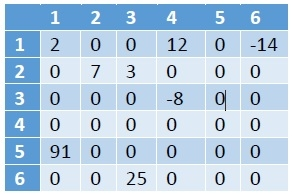# Check if a given matrix is sparse or not in C++

Here we will see how to check whether a matrix is sparse or not. A sparse matrix is a matrix where most of the elements are 0. The definition of a sparse matrix is, if the 2/3rd of the elements are 0, then the matrix is denoted as a sparse matrix. Here is the example of a sparse matrix.To check it, we will count the number of 0s in the matrix, then if that count is greater than 2/3rd of the total elements, then this is sparse.

## Example

Live Demo

#include <iostream>
#include <cmath>
#define MAX 5
using namespace std;
bool isSparseMatrix(int arr[][MAX], int m, int n) {
int counter = 0;
for (int i = 0; i < m; i++)
for (int j = 0; j <n; j++)
if (arr[i][j] == 0)
counter++;
return (counter > (2*(m * n) / 3));
}
int main() {
int matrix[MAX][MAX] = {
{0, 2, 0, 0, 0},
{8, 0, 0, 0, 0},
{0, 3, 0, 0, 0},
{0, 9, 0, 3, 0},
{0, 0, 0, 0, 4}
};
if(isSparseMatrix(matrix, MAX, MAX)){
cout << "This is sparse matrix";
} else {
cout << "This is not sparse matrix";
}
}

## Output

This is sparse matrix